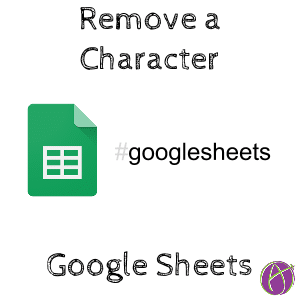#I sometimes have a need to extract part of word or text string when using a spreadsheet. For example if I have a hashtag in my spreadsheet #googlesheets and I want to create a clickable hyperlink, the link does not like the hashtag # symbol.

## =LEN()

The first formula you might want to use is =len() which counts the number of characters in a cell. For example if you have #googlesheets in cell B1 then using =len(B1) will return 13.

## =RIGHT()

The formula =right() returns the specified number of characters from the right. For example if #googlesheets is in cell B1 then =right(B1,3) returns ets.

## Together

Use these 2 formulas together (plus a little of your algebra skills). You want the number of characters minus the first character. So if #googlesheets is in cell B2 then =right(B2, len(B2)-1) will return googlesheets.This site uses Akismet to reduce spam. Learn how your comment data is processed.